# 一文读懂BiLSTM+CRF实现命名实体识别¶

BiLSTM + CRF是一种经典的命名实体识别（NER）模型方案，这在后续很多的模型improvment上都有启发性。如果你有了解NER任务的兴趣或者任务，或者完全出于对CRF的好奇，建议大家静心读一读这篇文章。

1. 一种实现NER的策略：BiLSTM+CRF

2. 回归CRF建模原理本身
2.1 线性CRF的定义
2.2 发射分数和转移分数
2.3 建模CRF的损失函数
2.4 单条路径的分数计算
2.5 全部路径的分数计算
2.6 CRF的Viterbi解码

## 1. 使用BiLSTM+CRF实现NER ¶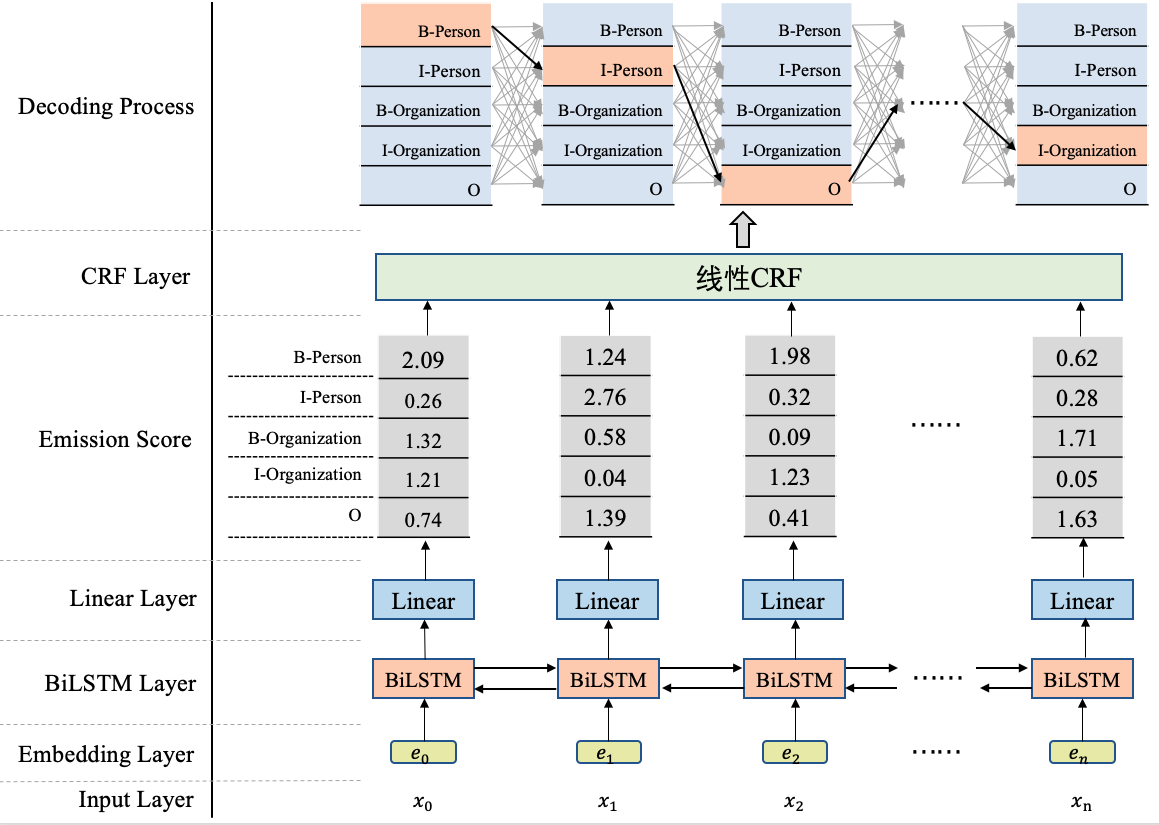• B-Person, I-Person, O, …, I-Organization

• B-Organization, I-Person, O, …, I-Person

• B-Organization, I-Organization, O, …, O

CRF的作用就是在所有可能的路径中，找出得出概率最大，效果最优的一条路径，那这个标签序列就是模型的输出。

## 2. 回归CRF建模原理本身¶

### 2.1 线性CRF的定义¶

$$X=[x_1, x_2, ..., x_n]，Y=[y_1, y_2, ..., y_n]$$ 均为线性链表示的随机变量序列，若在给定随机变量序列的$$X$$的条件下，随机变量序列$$Y$$的条件概率分布$$P(Y|X)$$构成条件随机场，即满足马尔可夫性:

\begin{split} \begin{align} P(y_i|X, y_{1},...,y_{i-1},y_{i+1},...,y_n) &= P(y_i|X,y_{i-1},y_{i+1}) \\ i &= 1,2,...,n (在i=1和n时只考虑单边) \end{align} \end{split}

1. 确保输入序列$$X$$和输出序列$$Y$$是线性序列

2. 每个标签$$y_i$$的产生，只与这些因素有关系：当前位置的输入$$x_i$$$$y_i$$直接相连的两个邻居$$y_{i-1}$$$$y_{i+1}$$，与其他的标签和输入没有关系。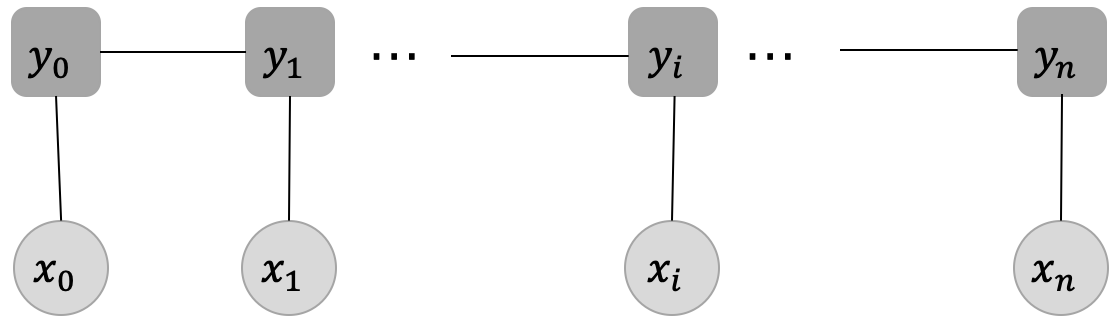### 2.2 发射分数和转移分数¶

#### 2.2.1 发射分数¶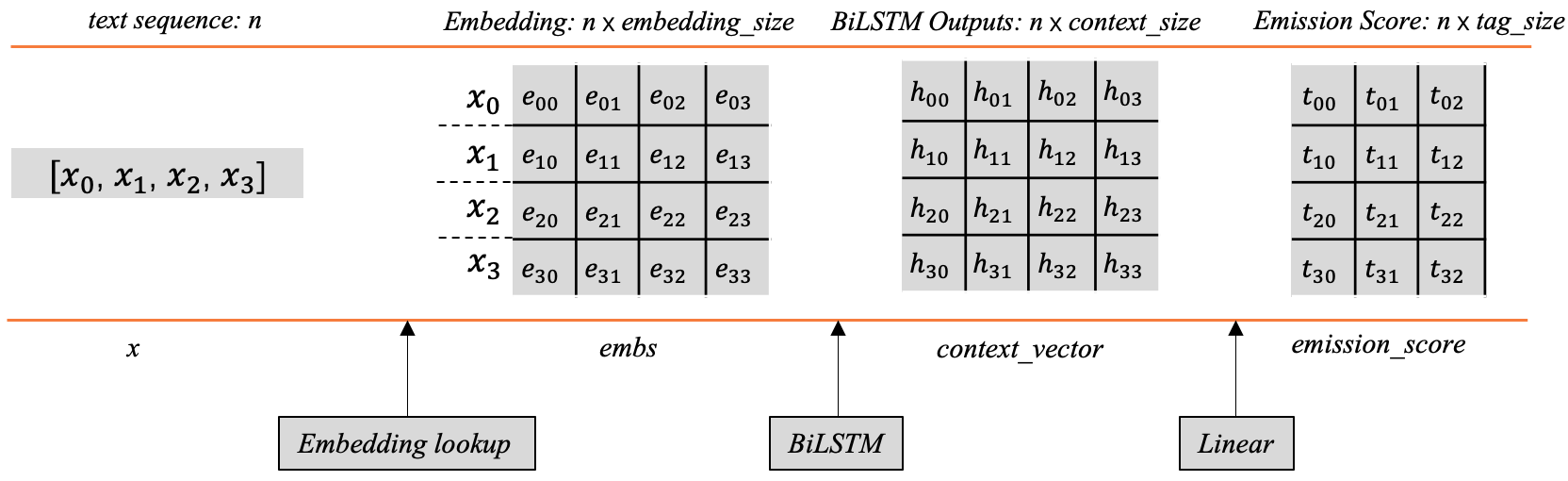#### 2.2.2 转移分数¶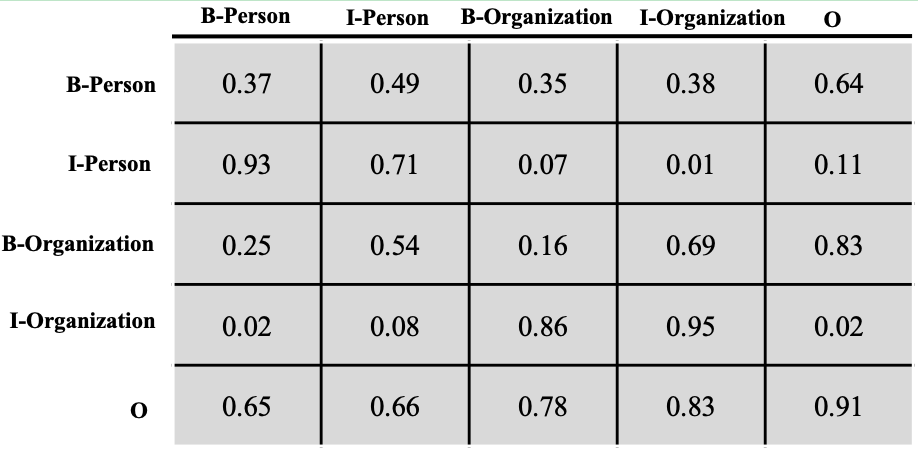$$Seq_t = T_{I-Person,B-Person} + T_{O,I-Person} + T_{O,O} + T_{O,B-Organization} + T_{B-Organization, I-Organization}$$

### 2.3 CRF建模的损失函数¶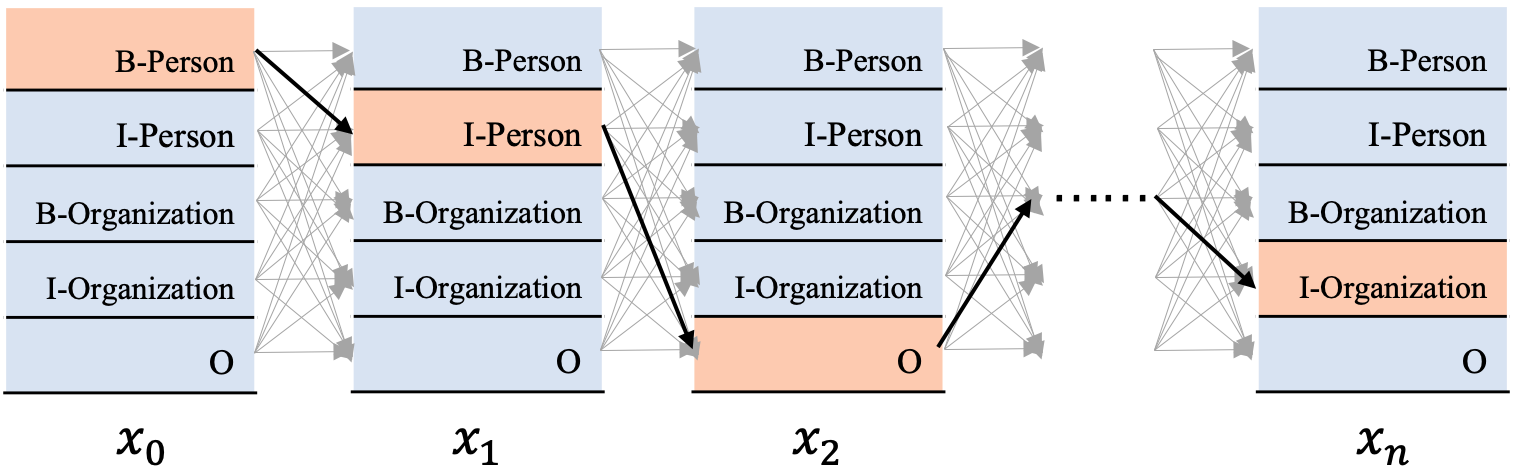$(y|x) = P(y_0,y_1,...,y_n|x_0,x_1,...,x_n)$

$P(S_i)=\frac{e^{S_i}}{\sum_j^{N}{e^{S_j}}}$

$P(S_{real})=\frac{e^{S_{real}}}{\sum_j^{N}{e^{S_j}}}$

$loss=-P(S_{real})=-\frac{e^{S_{real}}}{\sum_j^{N}{e^{S_j}}}$

\begin{split} \begin{align} loss &= -log \frac{e^{S_{real}}}{\sum_j^{N}{e^{S_j}}} \\ &= -(log(e^{S_{real}}) - log(\sum_j^{N}{e^{S_j}})) \\ &= log(\sum_j^{N}{e^{S_j}}) - log(e^{S_{real}}) \\ &= log(e^{S_1}+e^{S_2}+...+e^{S_N}) - S_{real} \\ \end{align} \end{split}

### 2.4 单条路径的分数计算¶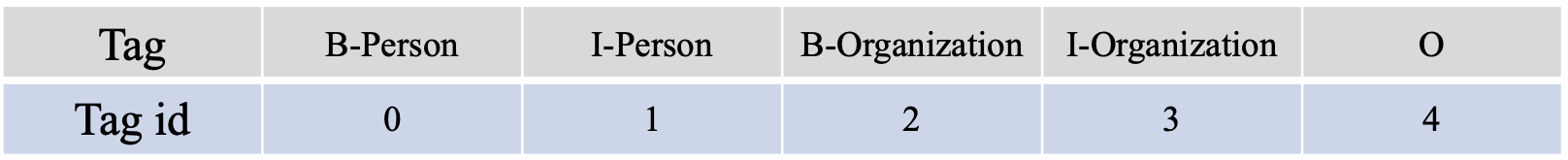$S_{real}=\sum_{i=0}^{n-1}{E_{i,y_i}} + \sum_{i=0}^{n-2}{T_{y_{i+1}, y_{i}}}$

### 2.5 全部路径的分数计算¶

2.3节中的损失函数包括两项，单条真实路径分数的计算和归一化项（如上所述，全部路径分数的$$log\_sum\_exp$$，为方便描述，后续直接将个归一化项描述为全部路径之和）的计算。这里你或许会问，现在知道了单条路径分数的计算方式，遍历一下所有的路径算个分数，不就可以轻松算出全部路径之和吗？是的，这在理论上是可行的。

$log(e^{S_1}+e^{S_2}+...+e^{S_N})$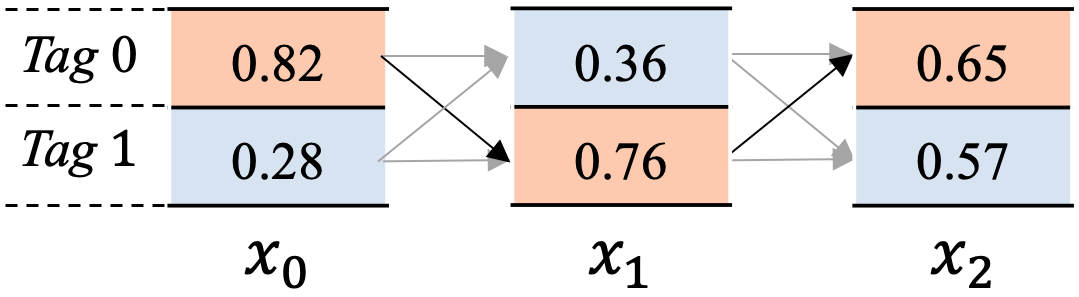$$emission_i=[x_{i0},x_{i1}]$$， 代表$$x_i$$位置的发射分数。

$$trans = \left[ \begin{matrix} t_{00} & t_{01}\\ t_{10} & t_{11} \end{matrix} \right]$$, 代表转移矩阵。

$$alpha_i=[s_{i0},s_{i1}]$$, 其中各个数值代表到当前位置$$x_i$$为止，以$$x_i$$位置相应标签结尾的路径分数之和。

$$x_2$$步为例，$$alpha_2=[s_{20},s_{21}]$$，其中$$s_{20}$$代表截止到$$x_2$$步骤为止，以标签$$Tag$$ 0 结尾所有的路径分数之和，$$s_{21}$$代表截止到$$x_2$$步骤为止，以标签$$Tag$$ 1结尾的所有路径分数之和。

• $$x_{00},x_{10},x_{20}$$

• $$x_{00},x_{11},x_{20}$$

• $$x_{01},x_{10},x_{20}$$

• $$x_{01},x_{11},x_{20}$$

$total_0 = log(e^{x_{00}}+e^{x_{01}})$

$\begin{split}emission_1 = \left[ \begin{matrix} x_{10} & x_{10}\\ x_{11} & x_{11} \end{matrix} \right] \end{split}$
$\begin{split}alpha_0 = \left[ \begin{matrix} x_{00} & x_{01}\\ x_{00} & x_{01} \end{matrix} \right] \end{split}$

\begin{split} \begin{align} scores &= alpha_0+trans+emission_1 \\ &= \left[ \begin{matrix} x_{00} & x_{01}\\ x_{00} & x_{01} \end{matrix} \right] + \left[ \begin{matrix} t_{00} & t_{01}\\ t_{10} & t_{11} \end{matrix} \right] + \left[ \begin{matrix} x_{10} & x_{10}\\ x_{11} & x_{11} \end{matrix} \right] \\ &= \left[ \begin{matrix} x_{00}+t_{00}+x_{10} & x_{01}+t_{01}+x_{10}\\ x_{00}+t_{10}+x_{11} & x_{01}+t_{11}+x_{11} \end{matrix} \right] \end{align} \end{split}

$$alpha_1 = [log(e^{x_{00}+t_{00}+x_{10}}+e^{x_{01}+t_{01}+x_{10}}), log(e^{x_{00}+t_{10}+x_{11}}+e^{x_{01}+t_{11}+x_{11}})]$$

\begin{split} \begin{align} total_1 &= log(e^{alpha_1} + e^{alpha_1}) \\ &=log(e^{log(e^{x_{00}+t_{00}+x_{10}}+e^{x_{01}+t_{01}+x_{10}})}+e^{log(e^{x_{00}+t_{10}+x_{11}}+e^{x_{01}+t_{11}+x_{11}})}) \\ &=log(e^{x_{00}+t_{00}+x_{10}}+e^{x_{01}+t_{01}+x_{10}} + e^{x_{00}+t_{10}+x_{11}}+e^{x_{01}+t_{11}+x_{11}}) \end{align} \end{split}

$\begin{split}emission_2 = \left[ \begin{matrix} x_{20} & x_{20}\\ x_{21} & x_{21} \end{matrix} \right] \end{split}$
$\begin{split}alpha_1 = \left[ \begin{matrix} log(e^{x_{00}+t_{00}+x_{10}}+e^{x_{01}+t_{01}+x_{10}}) & log(e^{x_{00}+t_{10}+x_{11}}+e^{x_{01}+t_{11}+x_{11}}) \\ log(e^{x_{00}+t_{00}+x_{10}}+e^{x_{01}+t_{01}+x_{10}}) & log(e^{x_{00}+t_{10}+x_{11}}+e^{x_{01}+t_{11}+x_{11}}) \end{matrix} \right] \end{split}$

\begin{split} \begin{align} scores &= alpha_1+trans+emission_2 \\ &= \left[ \begin{matrix} log(e^{x_{00}+t_{00}+x_{10}}+e^{x_{01}+t_{01}+x_{10}}) & log(e^{x_{00}+t_{10}+x_{11}}+e^{x_{01}+t_{11}+x_{11}}) \\ log(e^{x_{00}+t_{00}+x_{10}}+e^{x_{01}+t_{01}+x_{10}}) & log(e^{x_{00}+t_{10}+x_{11}}+e^{x_{01}+t_{11}+x_{11}}) \end{matrix} \right] + \left[ \begin{matrix} t_{00} & t_{01}\\ t_{10} & t_{11} \end{matrix} \right] + \left[ \begin{matrix} x_{20} & x_{20}\\ x_{21} & x_{21} \end{matrix} \right] \\ &= \left[ \begin{matrix} log(e^{x_{00}+t_{00}+x_{10}}+e^{x_{01}+t_{01}+x_{10}})+t_{00}+x_{20} & log(e^{x_{00}+t_{10}+x_{11}}+e^{x_{01}+t_{11}+x_{11}})+t_{01}+x_{20}\\ log(e^{x_{00}+t_{00}+x_{10}}+e^{x_{01}+t_{01}+x_{10}})+t_{10}+x_{21} & log(e^{x_{00}+t_{10}+x_{11}}+e^{x_{01}+t_{11}+x_{11}})+t_{11}+x_{21} \end{matrix} \right] \end{align} \end{split}

\begin{split} \begin{align} alpha_2 &= [log(e^{scores[0,0]} + e^{scores[0,1]}), log(e^{scores[1,0]} + e^{scores[1,1]})] \\ &=[log(e^{log(e^{x_{00}+t_{00}+x_{10}}+e^{x_{01}+t_{01}+x_{10}})+t_{00}+x_{20}} + e^{log(e^{x_{00}+t_{10}+x_{11}}+e^{x_{01}+t_{11}+x_{11}})+t_{01}+x_{20}}), log(e^{log(e^{x_{00}+t_{00}+x_{10}}+e^{x_{01}+t_{01}+x_{10}})+t_{10}+x_{21}} + e^{log(e^{x_{00}+t_{10}+x_{11}}+e^{x_{01}+t_{11}+x_{11}})+t_{11}+x_{21}})] \\ &=[log((e^{x_{00}+t_{00}+x_{10}}+e^{x_{01}+t_{01}+x_{10}})e^{t_{00}+x_{20}}+(e^{x_{00}+t_{10}+x_{11}}+e^{x_{01}+t_{11}+x_{11}})e^{t_{01}+x_{20}}), log((e^{x_{00}+t_{00}+x_{10}}+e^{x_{01}+t_{01}+x_{10}})e^{t_{10}+x_{21}}+(e^{x_{00}+t_{10}+x_{11}}+e^{x_{01}+t_{11}+x_{11}})e^{t_{11}+x_{21}})] \end{align} \end{split}

\begin{split} \begin{align} total_2 &= log(e^{alpha_2} + e^{alpha_2}) \\ &=log(e^{log((e^{x_{00}+t_{00}+x_{10}}+e^{x_{01}+t_{01}+x_{10}})e^{t_{00}+x_{20}}+(e^{x_{00}+t_{10}+x_{11}}+e^{x_{01}+t_{11}+x_{11}})e^{t_{01}+x_{20}})}+e^{log((e^{x_{00}+t_{00}+x_{10}}+e^{x_{01}+t_{01}+x_{10}})e^{t_{10}+x_{21}}+(e^{x_{00}+t_{10}+x_{11}}+e^{x_{01}+t_{11}+x_{11}})e^{t_{11}+x_{21}})}) \\ &=log((e^{x_{00}+t_{00}+x_{10}}+e^{x_{01}+t_{01}+x_{10}})e^{t_{00}+x_{20}}+(e^{x_{00}+t_{10}+x_{11}}+e^{x_{01}+t_{11}+x_{11}})e^{t_{01}+x_{20}}+ (e^{x_{00}+t_{00}+x_{10}}+e^{x_{01}+t_{01}+x_{10}})e^{t_{10}+x_{21}}+(e^{x_{00}+t_{10}+x_{11}}+e^{x_{01}+t_{11}+x_{11}})e^{t_{11}+x_{21}} ) \\ &= log(e^{x_{00}+t_{00}+x_{10}+t_{00}+x_{20}} + e^{x_{01}+t_{01}+x_{10}+t_{00}+x_{20}} + e^{x_{00}+t_{10}+x_{11}+t_{01}+x_{20}} + e^{x_{01}+t_{11}+x_{11}+t_{01}+x_{20}} + e^{x_{00}+t_{00}+x_{10}+t_{10}+x_{21}} + e^{x_{01}+t_{01}+x_{10}+t_{10}+x_{21}} + e^{x_{00}+t_{10}+x_{11}+t_{11}+x_{21}} + e^{x_{01}+t_{11}+x_{11}+t_{11}+x_{21}}) \end{align} \end{split}

### 2.6 CRF的Viterbi解码¶

$$emission_i=[x_{i0},x_{i1}]$$， 代表$$x_i$$位置的发射分数。

$$trans = \left[ \begin{matrix} t_{00} & t_{01}\\ t_{10} & t_{11} \end{matrix} \right]$$, 代表转移矩阵。

$$alpha_i=[s_{i0},s_{i1}]$$, 其中各个数值代表到当前位置$$x_i$$为止，以当前位置$$x_i$$相应标签结尾的路径中，取得最大分数的路径得分。

$$x_2$$位置为例，$$alpha_2=[s_{20},s_{21}]$$，其中$$s_{20}$$代表截止到$$x_2$$步骤为止，以标签$$Tag$$ 0 结尾所有的路径中得分最大的路径分数，$$s_{21}$$代表截止到$$x_2$$步骤为止，以标签$$Tag$$ 1结尾的所有路径中得分最大的路径分数。

$$beta_i = [p_{i0},p_{i1}]$$，其中各个数值代表到当前位置$$x_i$$为止，以当前位置$$x_i$$相应标签结尾的路径中，分数最大的那一条路径在前一个位置$$x_{i-1}$$的标签索引（每个标签对应的id号）。

$$x_2$$位置为例，$$beta_2 = [p_{20},p_{21}]$$，其中$$p_{20}$$代表代表截止到$$x_2$$步骤为止，以标签$$Tag$$ 0 结尾所有的路径中得分最大的那条路径在$$x_{i-1}$$位置的标签索引，同理$$p_{21}$$代表截止到$$x_2$$步骤为止，以标签$$Tag$$ 1结尾的最大路径在$$x_{i-1}$$位置的标签索引。

$\begin{split}emission_1 = \left[ \begin{matrix} x_{10} & x_{10}\\ x_{11} & x_{11} \end{matrix} \right] \end{split}$
$\begin{split}alpha_0 = \left[ \begin{matrix} x_{00} & x_{01}\\ x_{00} & x_{01} \end{matrix} \right] \end{split}$

\begin{split} \begin{align} scores &= alpha_0+trans+emission_1 \\ &= \left[ \begin{matrix} x_{00} & x_{01}\\ x_{00} & x_{01} \end{matrix} \right] + \left[ \begin{matrix} t_{00} & t_{01}\\ t_{10} & t_{11} \end{matrix} \right] + \left[ \begin{matrix} x_{10} & x_{10}\\ x_{11} & x_{11} \end{matrix} \right] \\ &= \left[ \begin{matrix} x_{00}+t_{00}+x_{10} & x_{01}+t_{01}+x_{10}\\ x_{00}+t_{10}+x_{11} & x_{01}+t_{11}+x_{11} \end{matrix} \right] \end{align} \end{split}

\begin{split} \begin{align} alpha_1 &= [max(scores[0,0], scores[0,1]), max(scores[1,0], scores[1,1])] \\ &= [max(x_{00}+t_{00}+x_{10}, x_{01}+t_{01}+x_{10}), max(x_{00}+t_{10}+x_{11}, x_{01}+t_{11}+x_{11})] \end{align} \end{split}

• $$x_{00}+t_{00}+x_{10} < x_{01}+t_{01}+x_{10}$$

• $$x_{00}+t_{10}+x_{11} > x_{01}+t_{11}+x_{11}$$

$\begin{split}emission_2 = \left[ \begin{matrix} x_{20} & x_{20}\\ x_{21} & x_{21} \end{matrix} \right] \end{split}$
$\begin{split}alpha_1 = \left[ \begin{matrix} max(x_{00}+t_{00}+x_{10}, x_{01}+t_{01}+x_{10}) & max(x_{00}+t_{10}+x_{11}, x_{01}+t_{11}+x_{11}) \\ max(x_{00}+t_{00}+x_{10}, x_{01}+t_{01}+x_{10}) & max(x_{00}+t_{10}+x_{11}, x_{01}+t_{11}+x_{11}) \end{matrix} \right] \end{split}$

\begin{split} \begin{align} scores &= alpha_1+trans+emission_2 \\ &= \left[ \begin{matrix} max(x_{00}+t_{00}+x_{10}, x_{01}+t_{01}+x_{10}) & max(x_{00}+t_{10}+x_{11}, x_{01}+t_{11}+x_{11}) \\ max(x_{00}+t_{00}+x_{10}, x_{01}+t_{01}+x_{10}) & max(x_{00}+t_{10}+x_{11}, x_{01}+t_{11}+x_{11}) \end{matrix} \right] + \left[ \begin{matrix} t_{00} & t_{01}\\ t_{10} & t_{11} \end{matrix} \right] + \left[ \begin{matrix} x_{20} & x_{20}\\ x_{21} & x_{21} \end{matrix} \right] \\ &= \left[ \begin{matrix} max(x_{00}+t_{00}+x_{10}, x_{01}+t_{01}+x_{10}) +t_{00}+x_{20} & max(x_{00}+t_{10}+x_{11}, x_{01}+t_{11}+x_{11})+t_{01}+x_{20}\\ max(x_{00}+t_{00}+x_{10}, x_{01}+t_{01}+x_{10})+t_{10}+x_{21} & max(x_{00}+t_{10}+x_{11}, x_{01}+t_{11}+x_{11}) +t_{11}+x_{21} \end{matrix} \right] \end{align} \end{split}

\begin{split} \begin{align} alpha_1 &= [max(scores[0,0], scores[0,1]), max(scores[1,0], scores[1,1])] \\ &= [max(max(x_{00}+t_{00}+x_{10}, x_{01}+t_{01}+x_{10}) +t_{00}+x_{20}, max(x_{00}+t_{10}+x_{11}, x_{01}+t_{11}+x_{11})+t_{01}+x_{20}), max(max(x_{00}+t_{00}+x_{10}, x_{01}+t_{01}+x_{10})+t_{10}+x_{21}, max(x_{00}+t_{10}+x_{11}, x_{01}+t_{11}+x_{11}) +t_{11}+x_{21} )] \end{align} \end{split}

• $$max(x_{00}+t_{00}+x_{10}, x_{01}+t_{01}+x_{10}) +t_{00}+x_{20} > max(x_{00}+t_{10}+x_{11}, x_{01}+t_{11}+x_{11})+t_{01}+x_{20})$$

• $$max(x_{00}+t_{00}+x_{10}, x_{01}+t_{01}+x_{10})+t_{10}+x_{21} < max(x_{00}+t_{10}+x_{11}, x_{01}+t_{11}+x_{11}) +t_{11}+x_{21} )$$

• $$x_{00}+t_{00}+x_{10} < x_{01}+t_{01}+x_{10}$$

• $$x_{00}+t_{10}+x_{11} > x_{01}+t_{11}+x_{11}$$

• $$x_{01}+t_{01}+x_{10}+t_{01}+x_{10} < x_{00}+t_{10}+x_{11}+t_{01}+x_{20}$$

• $$x_{01}+t_{01}+x_{10}+t_{10}+x_{21} > x_{00}+t_{10}+x_{11}+t_{11}+x_{21}$$

$$x_{00}+t_{10}+x_{11}+t_{01}+x_{20} > x_{01}+t_{01}+x_{10}+t_{10}+x_{21}$$

## 3. Reference¶

 邱锡鹏. 神经网络与深度学习[M]. 北京：机械工业出版社，2021.
 吴飞. 人工智能导论：模型与算法[M]. 北京：高等教育出版社，2020.
 CRF Layer on the Top of BiLSTM